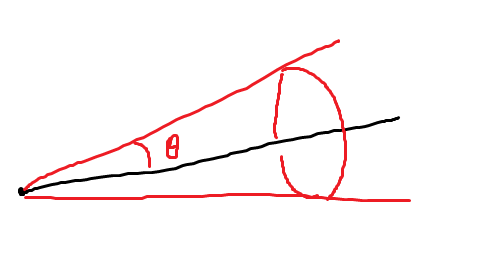# How do I change a parts velocity in the direction that the character is facing?

I want to push a part out in a random direction in front of the character within a certain radius, and that radius always stays the same based on what direction the character is facing.

It kind of does it, but the radius changes if the character rotates

``````local RandomNumber = math.random(-500,500) --Not a set number
local RandomNumber2 = math.random(100,500) --Not a set number
local RandomNumber3 = math.random(100,500) --Not a set number

Part.Velocity = script.Parent.CFrame.LookVector * Vector3.new(RandomNumber,RandomNumber2,RandomNumber3)
``````

Do you mean you want it to choose a random vector within a cone?

No

As an example I’ll use say a gun

With a gun, if the bullet shoots out in front of your character, I want to add in bullet spread so it’s random, but it has to stay in front of the character

My current issue is that it just doesn’t work properly. That radius changes based on the characters rotation/direction, and I don’t want that. You don’t want the gun to be shooting behind you if your character is looking a certain way.

i didn’t test this but maybe this is what you’re looking for?

``````local spread = 3
``````

spread variable isn’t a specific angle input

invalid argument #2 to ‘new’ (Vector3 expected, got CFrame)

You’re saying “radius”, but I think you want a cone. Radius isn’t quite specific enough in this context.

i.e. if your LookVector is the black line in this image, you want to pick some velocity in the red cone:I borrowed that image from this stackoverflow answer.

Here’s the top answer on that question translated into lua:

``````-- axis: center line of cone
-- angle: angle from center to edge of cone
-- returns: a random unit vector inside the cone, evenly distributed along the partial surface of a unit sphere.
local function RandomCone(axis: Vector3, angle: number)
local cosAngle = math.cos(angle)
local z = 1 - math.random()*(1 - cosAngle)
local phi = math.random()*math.pi*2
local r = math.sqrt(1 - z*z)
local x = r * math.cos(phi)
local y = r * math.sin(phi)
local vec = Vector3.new(x, y, z)
if axis.Z > 0.9999 then
return vec
elseif axis.Z < -0.9999 then
return -vec
end
local orth = Vector3.zAxis:Cross(axis)
local rot = math.acos(axis:Dot(Vector3.zAxis))
return CFrame.fromAxisAngle(orth, rot) * vec
end
``````

You’d use it like

``````-- angle of the cone, i.e. the largest angle off the firing axis you could be

-- speed of the bullet (can also be random if you want)
local SPEED = 100

part.AssemblyLinearVelocity = RandomCone(script.Parent.CFrame.LookVector, SPREAD) * SPEED
``````

Haven’t tested this yet. If this is not what you’re looking for please elaborate a bit more7 Likes

This is what I am looking for, but there are a few errors, so I can’t use the script.

The ‘dir’ and ‘Vector’ are undefined. Can you please test and fix this?

Fixed up the typos and edited my reply.

You didn’t? It’s still the same as before

1 Like

My bad. Edited for real this timeThanks a bunch!!! You’re the best